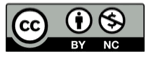# Scikit-learn 学习笔记（3）监督学习的例子

2022-08-04## 监督学习要解决的问题## 分类问题的例子

 import numpy as np
from sklearn import datasets

iris_X, iris_y = datasets.load_iris(return_X_y=True)
print(np.unique(iris_y))


[0 1 2]


## K-最近邻算法

scikit-learn 库里的每一个监督估计器都有一个 .fit(X, y) 方法函数和一个 predict(X) 方法函数。.fit(X, y) 用于拟合数据，predict(X) 用于预测 y

scikit-learn 库，y 向量的成员是整数或者字符串。

## 捐助本站